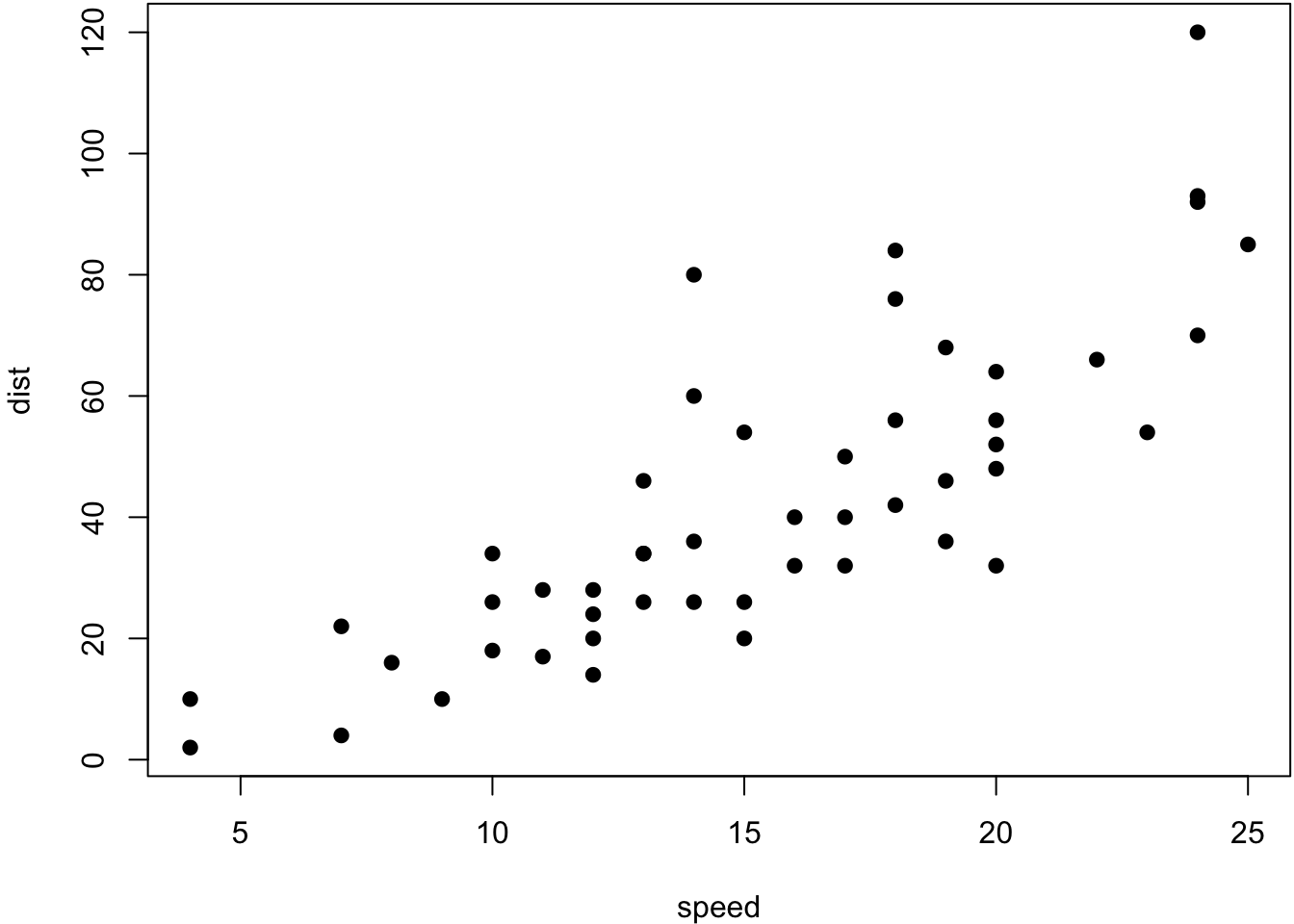# Chapter 2 Syntax

## 2.1 Basic

You can make text italic by surrounding it with underscores or asterisks, e.g., text or text. For bold text, use two underscores (text) or asterisks (text). Text surrounded by ~ will be converted to a subscript (e.g., H2SO4 renders H2SO4), and similarly, two carets (^) produce a superscript (e.g., Fe2+ renders Fe2+). To mark text as inline code, use a pair of backticks, e.g., code.3 Small caps can be produced by the HTML tag span, e.g., Small Caps renders Small Caps. Links are created using text, e.g., RStudio. Footnotes are put inside the square brackets after a caret 1, e.g., 2.

Blockquotes are possible

“Rishi likes blockquotes a lot. If anyone thinks blockquotes are useless, they are incorrect and should rethink their priorities and go do something else

— “Rishi Goutam”

This is a code block with no language
specified at all
This is also a code block

## 2.3 Math

$e^{\pi i} + 1 = 0$ $X = \begin{bmatrix}1 & x_{1}\\ 1 & x_{2}\\ 1 & x_{3} \end{bmatrix}$

$\begin{vmatrix}a & b\\ c & d \end{vmatrix}=ad-bc$

We can number an equation $\begin{equation} e^{\pi i} + 1 = 0 \tag{2.1} \end{equation}$

and refer to it (2.1)

## 2.4 Special custom blocks

Bookdown provides some special handling for custom blocks, such as theorem and example. See here for the full list

Theorem 2.1 (Pythagorean theorem) For a right triangle, if $$c$$ denotes the length of the hypotenuse and $$a$$ and $$b$$ denote the lengths of the other two sides, we have $a^2 + b^2 = c^2$

We can refer to a named theorem. Remember trig theorem 2.1

## 2.5 Tables

We can have tables

Table 2.1: A table of the first 10 rows of the mtcars data.
mpg cyl disp hp drat wt qsec vs
Mazda RX4 21.0 6 160.0 110 3.90 2.620 16.46 0
Mazda RX4 Wag 21.0 6 160.0 110 3.90 2.875 17.02 0
Datsun 710 22.8 4 108.0 93 3.85 2.320 18.61 1
Hornet 4 Drive 21.4 6 258.0 110 3.08 3.215 19.44 1
Hornet Sportabout 18.7 8 360.0 175 3.15 3.440 17.02 0
Valiant 18.1 6 225.0 105 2.76 3.460 20.22 1
Duster 360 14.3 8 360.0 245 3.21 3.570 15.84 0
Merc 240D 24.4 4 146.7 62 3.69 3.190 20.00 1
Merc 230 22.8 4 140.8 95 3.92 3.150 22.90 1
Merc 280 19.2 6 167.6 123 3.92 3.440 18.30 1
Table 2.2: A Tale of Two Tables.
Sepal.Length Sepal.Width
5.1 3.5
4.9 3.0
4.7 3.2
mpg cyl disp
Mazda RX4 21.0 6 160
Mazda RX4 Wag 21.0 6 160
Datsun 710 22.8 4 108
Hornet 4 Drive 21.4 6 258

## 2.6 Figures

par(mar = c(4, 4, .1, .1))
plot(cars, pch = 19)Figure 2.1: A figure example with a relative width 70%.

knitr::include_graphics(rep('memoji.png'))Figure 2.2: Memoji of Rishi Goutam

## 2.7 HTML Widgets

See htmlwidgets

We can also use JavaScript libraries for rich data visualizations. Install the below for outputting to non-HTML files.

install.packages("webshot")
webshot::install_phantomjs()

We use the DT package to show the iris dataset:

DT::datatable(iris)

Figure 2.3: A table widget rendered via the DT package.

1. This is a footnote.↩︎